# Logic Gates and its Types

## Definition of Logic Gates

A digital circuit that takes in one or more inputs and produces a single output is called a logic gate. Both the input and the output are digital signals.

Or you can say that a logic gate is an electronic circuit or device that makes the logical decision.

The gate can be constructed from:

• Diodes
• Transistor
• Register

## Types of Logic Gates

There are basically seven types of logic gates available that are categorized into three categories:

1. Basic Gates
2. Universal Gates
3. Special Purpose Gates

Let's go over each of the aforementioned logic gate types in detail, including their definitions, boolean equations, and truth tables.

### AND Gate

An AND gate is a logic circuit that consists of two or more inputs and only one output.

In an AND gate, the output is high if and only if all the inputs to the gate are high. Otherwise, the output will be low. Here, high indicates 1 and low indicates 0. In a digital circuit, we only talk about 0 and 1.

#### Symbol of the AND Gate

Here is the symbol for the two-input AND gate: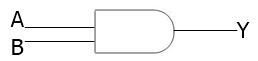Here, A and B are the two inputs, and Y is the output.

#### AND Gate Boolean Equation

Here is the boolean equation of a two-input AND gate:

`Y = A.B`

#### AND Gate Truth Table

The table given below represents or shows the truth table of a two-input AND gate:

Input Output
A B Y
0 0 0
0 1 0
1 0 0
1 1 1

### OR Gate

An OR gate is a logic circuit with two or more inputs and only one output.

The function of an OR gate can be expressed as follows: if either of the two inputs is true, then the output will be true; otherwise, the output will be false. Here, true means 1 and false means 0.

#### Symbol of the OR Gate

Below is the symbol for the two-input OR gate: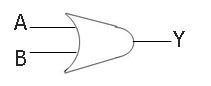#### Boolean Expression for OR Gate

The boolean expression of the two-input OR gate will be:

`Y = A + B`

#### OR Gate Truth Table

The table given below shows the truth table of an OR gate:

Input Output
A B Y
0 0 0
0 1 1
1 0 1
1 1 1

### NOT Gate

A NOT gate is used to reverse the input, which means that when the input is true, the output will not be true, implying that the output is false.

#### Symbol of the NOT Gate

Here is the symbol used for the NOT gate: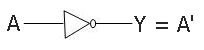#### NOT Gate Boolean Expression

The boolean expression of the NOT gate is:

`Y = A'`

#### The Truth Table of the NOT Gate

The NOT gate's truth table is as follows:

Input Output
A Y
0 1
1 0

### NAND Gate

The NAND gate, or NOT-AND gate, is made up of two gates. The first one is an AND gate followed by a NOT gate.

We can use the NAND gate whenever we want to reverse the value of the AND gate's output.

#### Symbol of the NAND Gate

The symbol of a two-input NAND gate is given below: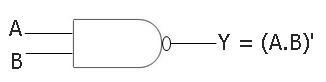#### Boolean Expression of the NAND Gate

The boolean expression of a two-input NAND gate will be:

`Y = (A.B)'`

As we can see from the above expression, it reverses the value of the AND gate output's value.

#### The NAND Gate Truth Table

The table given below shows the truth table of a two-input NAND gate:

Input Output
A B Y
0 0 1
0 1 1
1 0 1
1 1 0

### NOR Gate

The NOR gate is a little bit similar to the NAND gate. OR gate is used instead of AND gate in this case.Therefore, we can say that a NOR gate is a combination of an OR gate followed by a NOT gate.

This gate is used to reverse the value of the OR gate's output. It is abbreviated as the NOT-OR gate.

#### Symbol of the NOR Gate

The symbol used for a two-input NOR gate is: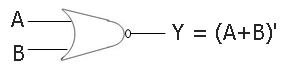#### Boolean Expression for NOR Gate

The boolean expression of a two-input NOR gate will be:

`Y = (A+B)'`

#### NOR Gate's Truth Table

The table given below shows the truth table of a two-input NOR gate or NOT-OR gate:

Input Output
A B Y
0 0 1
0 1 0
1 0 0
1 1 0

### XOR Gate

The XOR gate can also be called the EX-OR gate or EOR gate and is pronounced as the Exclusive OR gate.

An exclusive OR gate can have two or more input terminals and only one output terminal.

#### The XOR Gate's symbol

Here is the symbol given for the two-input XOR gate: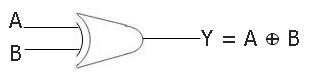#### Boolean Expression of the XOR Gate

The boolean expression of a two-input XOR gate is:

`Y = A ⊕ B`

#### The XOR Gate Truth Table

The table given below shows the truth table of a two-input XOR gate:

Input Output
A B Y
0 0 0
0 1 1
1 0 1
1 1 0

### XNOR Gate

XNOR, EX-NOR, or ENOR gates are pronounced as Exclusive NOR or Exclusive NOT-OR gates. Or, to put it another way, exclusive NOR means NOT Exclusive OR. It means it is the reverse of an XOR gate.

This gate is used to reverse the value of the XOR gate.

#### XNOR Gate Symbol

Below is the symbol of a two-input XNOR gate:#### XNOR Gate Boolean Expression

Here is the boolean equation of a two-input XNOR gate:

`Y = (A ⊕ B)'`

#### The Truth Table of XNOR Gate

The table given below shows the truth table of a two-input XNOR gate:

Input Output
A B Y
0 0 1
0 1 0
1 0 0
1 1 1

Computer Fundamentals Quiz

« Previous Tutorial Next Tutorial »